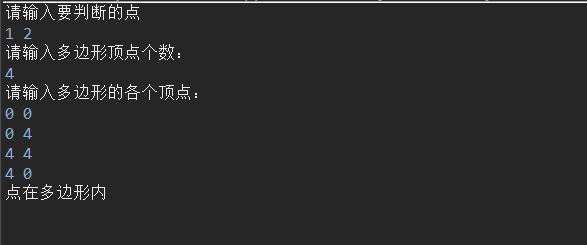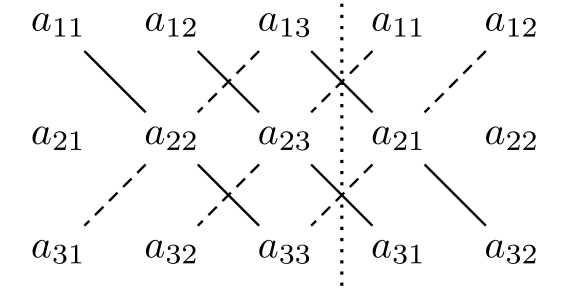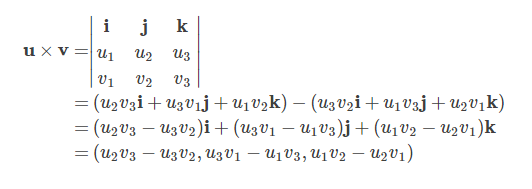• 需求：给定一个二维平面平面上有 n 个，求最多有多少在同一条直线上。 分析思路： 1、将所有二维坐标化，即定义出所有的x，y坐标值 2、遍历出所有取出两的情况(不考虑先后顺序)，根据任意两确定一...
需求：给定一个二维平面，平面上有 n 个点，求最多有多少个点在同一条直线上。
分析思路： 1、将所有点二维坐标化，即定义出所有点的x，y坐标值 2、遍历出所有取出两点的情况(不考虑先后顺序)，根据任意两点都确定一条直线，直线参数为k斜率，b与y轴交点的纵坐标（此时x=0），将他们放入一个列表中 3、将所有直线放入一个集合并完成去重操作，增加直线的第三个参数n=0用于第四步判断每条直线上有几个点 4、将所有点遍历并判断是否在集合中的直线上，若在直线上，则直线对应的n加1 5、遍历所有代表直线的列表，取出n最大的直线其n值就是最多有n个点在此条直线上
def line(point1, point2):
#定义一个函数通过两点来计算出对应直线的参数，
#传入的参数point1、point2都是列表
try:
y1 = point1
y2 = point2
x1 = point1
x2 = point2
#根据列表对应下标取出x、y值
k = (y2-y1)/(x2-x1)
#根据x、y值计算出斜率，当斜率无穷大时报错，进入except
b = y1-k*x1
#计算出b
return [k, b,0]
#返回直线参数，第三个参数为0，用来后面的计数
except Exception:
return ["+8", y1, 0]
#当报错时意味着斜率为无穷大，我们用"+8"代替

def judge_in(point_in, line_in):
#用来判断点是否在直线上，若在则返回True，
#若不在则返回False
x_in = point_in
y_in = point_in
k_in = line_in
b_in = line_in
if k_in == "+8":
#当斜率无穷大时，单独判断
if b_in == y_in:
return True
else:
return False
elif y_in == x_in*k_in+b_in:
return True
else:
return False

"""可以改变下方列表中点的参数"""
point_list = [[1, 1], [3, 2], [5, 3], [4, 1], [2, 3], [1, 4]]
#给出一个包含几个点的列表

# point_list = [[1,1],[2,2],[3,3]]
line_list = []
#新建一个用来接收直线的空列表
new_list = []
#直线去重后加入此列表
for i in range(len(point_list)):
for j in range(i+1, len(point_list)):
#通过双层的for循环给出所有两个点的组合
line_s = line(point_list[i], point_list[j])
#利用函数求出直线的前两个参数
line_list.append(line_s)

print(line_list)
#得到的是一组有重复参数的直线
for k in line_list:
if k not in new_list:
#去重
new_list.append(k)
for m in point_list:
for n in new_list:
#遍历所有点和线，判断点是否在线上，
#若在则直线第三个用来计数的参数加1
if judge_in(m, n):
n += 1
print(new_list)
#输出去重完毕后的列表，再经过一次遍历即可找出最多点所在的直线

展开全文• 　对每组测试数据输出一个整数，占一行，在指定定坐标范围内指定平面上有多少个整数，若输入的三个不能确定一个平面，直接输出"-1"。 Sample Input 0 0 0 0 1 0 0 0 1 -2 2 -2 2 -2 2 1 1 1 2 2 2 1 1 ...Golang erlang r语言
• 我拥有1000经纬度的坐标，我想判断这些多少在北京丰台区，如何去判断 求思路
• 判断一个点是否在一个平面内 射线法 判断：若从一点发出的射线穿越过平面内多边形的的个数为偶数，则在多边形外，若为奇数，则在多边形内。 做法：从该取向右的一条射线，遍历多边形的每一条边，判断射线与...
判断一个点是否在一个平面内
射线法 判断：若从一点发出的射线穿越过平面内多边形的点的个数为偶数，则点在多边形外，若为奇数，则点在多边形内。 做法：从该点取向右的一条射线，遍历多边形的每一条边，判断射线与边的交点个数（0或1,重合情况另外处理），最后取和，判断奇偶。 那么，如何判断射线与边的交点个数？ 1.点就在边上，易于判断 2.点不在边上，射线与边重合，直接判断点不在多边形内 3.比较点与边的端点的y值，若点的y值不在两个端点的y值之间， 那么不再继续，比较下一条边。为什么？我说了，取的是一条水平向右的射线，若点的y在不在两个端点的y值之间，那么必然是没有交点的。
Java代码如下：
import java.util.*;
public class ryamethod{
static class Point{
float x;
float y;

Point(){}

Point(float x, float y){
this.x = x;
this.y = y;
}
}

public static boolean ray(Point p, List<Point> poly) {
float nx = p.x; float ny = p.y;

int cnt = 0; //计算射线穿过多边形的点的数目

int len = poly.size();
for(int i = 0, j = len - 1; i < len; j = i, ++i) {
float bx = poly.get(i).x; float by = poly.get(i).y;
float ux = poly.get(j).x; float uy = poly.get(j).y;

//点与多边形顶点重合
if((nx == bx && ny == by) || (nx == ux && nx == uy)) {
return true;
}

if((by < ny && uy >= ny) || (by >= ny && uy < ny)) {
//边上与点的坐标y相同的x坐标
float x = bx + (ny - by) * (ux - bx) / (uy - by);

//点在多边形的边上
if(x == nx) {
return true;
}

if(x > nx) {
cnt += 1;
}
}
}
if(cnt % 2 == 1) {
return true;
}
else {
return false;
}
}

public static void main(String[] args) {
Scanner sc = new Scanner(System.in);
System.out.println("请输入要判断的点");

float x , y;
x = sc.nextFloat();
y = sc.nextFloat();
Point p = new Point(x, y);
System.out.println("请输入多边形顶点个数：");
int n = sc.nextInt();
System.out.println("请输入多边形的各个顶点：");
List<Point> poly = new ArrayList<>();
for(int i = 0; i < n; ++i) {

x = sc.nextFloat();
y = sc.nextFloat();
Point pot = new Point(x, y);
}
boolean flag = rayCasting(p, poly);
if(flag == true) {
System.out.println("点在多边形内");
}else {
System.out.println("点不在多边形内");
}
}
}展开全文• # 到两端点所确定的直线的距离　classic formula is: # # d = [(x2-x1)*(y1-y)-(x1-x)*(y2-y1)] / sqrt((x2-x1)**2 + (y2-y1)**2) # xD = (x2 - x1) yD = (y2 - y1) detaX = x1 - X detaY = y1 - Y...
    # 点到两个端点所确定的直线的距离　classic formula is:
# # d = [(x2-x1)*(y1-y)-(x1-x)*(y2-y1)] / sqrt((x2-x1)**2 + (y2-y1)**2)
#
xD = (x2 - x1)
yD = (y2 - y1)
detaX = x1 - X
detaY = y1 - Y
norm2 = sqrt(xD ** 2 + yD ** 2)  # 注意norm2是一个数而不是numpy数组,因为xD, yD都是一个数。单个数字运算math比numpy快
b = thre
dist = xD * detaY - detaX * yD  # 常数与numpy数组(X,Y是坐标数组）的运算，broadcast
dist /= norm2
dist = np.abs(dist)
ratiox = np.abs(detaX / (xD + 1e-8))
ratioy = np.abs(detaY / (yD + 1e-8))
ratio = np.where(ratiox < ratioy, ratiox, ratioy)
ratio = np.where(ratio > 1, 1, ratio)  # 不用　np.ones_like(ratio)也可以正常运行，并且会快一点点
ratio = np.where(ratio > 0.5, 1 - ratio, ratio)
oncurve_dist = b * np.sqrt(1 - np.square(ratio * 2))  # oncurve_dist计算的是椭圆边界上的点到长轴的垂直距离

sigma = thre  # todo: sigma of PAF 对于PAF的分布，设其标准差为多少最合适呢

guass_dist[dist >= b] = 0

return guass_dist

展开全文• 如何在vtk中根据三点提取一个切面
如何在vtk中根据三点提取一个切面，一直知道根据三点就能表示一个面，但是怎么在vtk中实现，对于我这种菜鸟真是要想好久。首先说说现在的思路，知道vtkImagepalne中可以根据一个点和一个面法向量获得一个面。现在我有三个点，需要获得一个面法向量，那么需要通过叉乘得到，再然后根据一个点和得到的面法向量显示一个切面。
之前看过vtkresliceImage得到的切面方法，感觉对变换的理解很差，尤其对Matrix（矩阵基本变换）的理解又不那么到位：  double center;  center = origin + spacing * 0.5 * (extent + extent);  center = origin + spacing * 0.5 * (extent + extent);  center = origin + spacing * 0.5 * (extent + extent)；  static double axialElements = {  1, 0, 0, 0,  0, 1, 0, 0,  0, 0, 1, 0,  0, 0, 0, 1 };  vtkSmartPointer<vtkMatrix4x4> resliceAxes =  vtkSmartPointer<vtkMatrix4x4>::New();  resliceAxes->DeepCopy(axialElements);  resliceAxes->SetElement(0, 3, center);  resliceAxes->SetElement(1, 3, center);  resliceAxes->SetElement(2, 3, center);  根据代码的理解，vtkMatrix4x4应该包含一个面和一个点，但是这个面是由static double axialElements = {  1, 0, 0, 0,  0, 1, 0, 0,  0, 0, 1, 0,  0, 0, 0, 1 };    决定的，根据定义，这个矩阵左上方3x3的矩阵是X、Y、Z方向的三个矢量，第四列三个做透视变换用的，根据水灵书中提的，这里好像是切面的坐标系原点（我没有看过透视变换的相关信息，不太了解）。第四行左三个别控制其在x轴y轴z轴上的平移单位.，第四个表示缩放比例。
再理解一下叉乘，叉乘（cross product）或者外积，它的计算结果是一个向量而非标量。叉积所在的向量与参与运算的两个向量都正交，也即正交于原来的两个向两边所决定的平面，也即两向量所决定的平面的法向量可通过计算叉积的方式得以确定。当参与运算的两向量是平行的两个向量时，得到的叉积为0，也即可通过计算叉积的方式判断两向量是否平行。  代数定义  二维时  x×y=x1y2−x2y1  三维时这里写图片描述  根据如图的计算方法可得：几何定义
x×y=∥x∥∥y∥sinθn⃗  n⃗ 表示叉积方向上的单位向量。
中学知识告诉我们三角形的面积计算公式为：
S=∥x∥∥y∥sinθ2=∥z∥h2
其中θ表示的是x,y之间的夹角，由以上两个公式我们可得到三角形的高或者点到其所对的边的距离，也即点到直线的距离，的计算公式：  h=∥x×y∥/∥z∥
double operator*(const Vec& x, const Vec& y)
{
assert(x.size() == y.size());       // #include <cassert>
double sum = 0.;
for (size_t i = 0; i < x.size(); ++i)
sum += x[i]*y[i];
return sum;
}

// 三维的情况
Vec operator^(const Vec& x, const Vec& y)
{
assert(x.size() == y.size() && x.size() == 3);
return Vec{x*y-x*y,
x*y-x*y,
x*y-x*y};
// uniform initialization, C++11新特性
}

// 二维就姑且返回其模长吧
double twoDCrossProd(const Vec& x, const Vec& y)
{
return x*y-x*y;
}
现在贴一段代码[C++实现叉乘]，并感谢作者提供。(http://blog.sina.com.cn/s/blog_4e24d9c50100ttvz.html)
#include <iostream>
#define M 3
#define N 4
#define P 3
using namespace std;
void getmt(int* m,int r,int c)              //获取矩阵
{
for(int i=0;i<r;++i)
{
for(int j=0;j<c;++j) cin>>*(m+i*c+j);
}
}
void showmt(const int* m,int r,int c)      //显示矩阵
{
for(int i=0;i<r;++i)
{
for(int j=0;j<c;++j) cout<<*(m+i*c+j)<<"\t";
cout<<endl;
}
}
void mmt(const int* m1,const int* m2,int* m3,int m,int n,int p)
{
for(int i=0;i<m;++i)                 //叉乘运算
{
for(int j=0;j<p;++j)
{
for(int k=0;k<n;++k)
{
(*(m3+i*p+j))+=(*(m1+i*n+k))*(*(m2+k*p+j));
}
}
}
}
int main(int argc, char *argv[])
{
int m1[M][N];
int m2[N][P];
int m3[M][P];
memset(m3, 0, sizeof(m3));    //注意不能用int m3[M][P]={0};来初始化为0,这样可能并没有将m3中的元素赋0
cout<<"为矩阵1输入"<<M<<"*"<<N<<"个数："<<endl;
getmt(&m1,M,N);        //获取矩阵m1
cout<<"为矩阵2输入"<<N<<"*"<<P<<"个数："<<endl;
getmt(&m2,N,P);        //获取矩阵m2
mmt(&m1,&m2,&m3,M,N,P);   //叉乘运算
cout<<"矩阵1："<<endl;
showmt(&m1,M,N);
cout<<"矩阵2："<<endl;
showmt(&m2,N,P);
cout<<"结果："<<endl;
showmt(&m3,M,P);
system("PAUSE");
return EXIT_SUCCESS;
}
搜了一下在图形学中表示向量的方法，看来这里面学问挺多的，贴两个实用的帖子，感谢作者提供宝贵的知识图形学中向量操作类、图像学中向量说明。
vtkImagePlaneWidget中不能直接设置SetNormal和SetCenter，只能设置初始点位置，那么这样就需要写一个类来继承vtkImagePlaneWidget，并且添加这两个功能。最后，继承了vtkPlaneSource中SetNormal和SetCenter这两个功能，可以提取经过三个点的切面：
    ImagePlaneWidget *mplanewidget = ImagePlaneWidget::New();
mplanewidget->SetInteractor(iren);
mplanewidget->SetPicker(picker); //定义 内部选择器
mplanewidget->SetTexturePlaneProperty(ipwProp);//给切片设定纹理属性
mplanewidget->TextureInterpolateOff();
mplanewidget->GetPlaneProperty()->SetColor(1, 0, 0); //设置平面属性
mplanewidget->SetResliceInterpolateToLinear();//差值方法
//mplanewidget->RestrictPlaneToVolumeOn(); //确保平面在容器范围之内
mplanewidget->SetNormal(mnormalize);  //设定切面法向方向
mplanewidget->SetOrigin(moriginpoint); //设定切面的初始点位置
mplanewidget->SetCenter(moriginpoint);  //设定切面所在位置中心
mplanewidget->On();
mplanewidget->InteractionOn();
//mplanewidget->PlaceWidget(polyData->GetBounds());//获得polydata中的边界，最大最小值
展开全文vtk
• 平面上N个点，每两个点确定一条直线， 求出斜率最大的那条直线所通过的两个点（斜率不存在的情况不考虑）。时间效率越高越好。 平面上N个点，每两个点确定一条直线，求出斜率最大的那条直线所通过的...
• 采用射线法就可以判断一个点是否在多边形内， 只需从出发向右侧水平做出一条射线，如果跟多边形交点个数为奇数，则在多边形内，否则在多边形外。看一张图就可以看懂啦 图片来自：...多边形 几何 python acm
• 平面上N个点，没两个点确定一条直线，求出斜率最大的那条直线所通过的两个点（斜率不存在的情况不考虑）。时间效率越高越好。 先把N个点按x排序。 斜率k最大值为max(斜率(point[i],point[i+1])) 0 复杂度...
• 问题： 平面上N个点，每两个点之间可以确定一条直线，求出最大斜率。时间效率越高越好。 方法： 1. 先把N个点按x排序。2. 斜率k最大值为max(斜率(point[i],point[i+1])) 0 <=i <n-2。 3. 复杂度Nlog(N) ...
• 生成两个点，这算子在Halcon三维中常用。 讲连接成线 dev_set_line_width(2) *画 dev_set_color('red') gen_cross_contour_xld(Cross1, 90, 103, 16, 0.785398) gen_cross_contour_xld(Cross2, 377, 341...三维重建
• 给定三维空间里的任意三个确定一个平面方程Ax+By+Cz+D=0的求解过程及伪代码的实现 其思想就是 1.先求解该平面的法向量n 2.由法式将其中的任意一点代入公式即可以求得平面方程Ax+By+Cz+D=0的系数ABCD 3.伪代码...
• 不同A,B确定一条直线，AB相同返回的值全0 直线方程：Ax+By+c=0 A = y2 - y1; B = x1 - x2; C = -Ax1 - By1 = x2y1 - x1y2; 证明之后补上； Line LineMake(Point A, Point B) { Line l; l.A = B.y - A.y; l.B...
• 因此，给定平面上的一点和垂直于该平面一个非零向量，平面确定了。 这就是所谓的法式方程的基础。 (1)法向量： 任意垂直与一个平面的向量被称为法向量。 法向量有无数个。 (2)平面法式方程： ...数学
• [正常，d] = plot_line(p1, p2, p3) 其中， p1 = [xyz] 类似地，所有其他都以相同的模式传递。 如果假设平面的 eq 为： ax + by + cz = d; 此函数以 3 个点作为输入，返回 [abc] 和 dmatlab
• python实现平面的距离 目录 python实现平面的距离 1.三点定面 2.到面的距离 3.python实现到面的距离 ...空间上任意三个不共线的，可以确定一个平面，三点定面的例子： 2.到面的距离 ...
• Sceneform 框架下通过三个绘制平面（Plane） 两个点确定一条直线，三个点确定一个平面，绘制直线的比较好处理，凭空添加平面就很少见了，这篇文章就是基于这个需求做的 ...SceneForm
• 比如我要在原有的马鞍面上添加一个与X-Z平面平行的平面，例如y=-5这个平面。 下图是origin2017的主界面 在快捷键那一栏中有一个名字为New 3D Plot的下拉菜单，如下图所示，选择New 3D Parametric Plot 选项 接着...三维图
• 假设在平面内,有一个三角形,边长分别为a、b、c 三角形的面积S可由以下公式求得： S=√[p(p-a)(p-b)(p-c)] 而公式里的p为半周长：p=(a+b+c)/2 解析几何 设三点坐标，A(a,b),B(c,d),C(e,f)，则三角形面积为 S=|ad＋...
• 然后对属于同一斜率的多对，当成幅图，求取连通分支数。这里对对属于同一斜率的多对，当成幅图，求取连通分支数解释一下： 假设对于斜率k=1.0，有点对 (1,1) ,(2,2) (2,2),(3,3) (3,3,),(1,1) (4,3...
• 一个平面通常是由一个平面法线向量，如v1(1,2,3)，和到原点(0,0,0)到平面的距离确定的。 当然数学中还有其他定义方法，但是DirectX和OpenGL都是使用这种定义方法的。这样定义的平面叫一般平面，其实是由法式...
• 到面的距离一般还说时最短距离，但一个平面一般是有界限的，所以需要先把一个平面用截距式方程显示为： Ax+By+Cz+D=0 借用这张图来表示： 可以看到要求d（最短距离）需要知道q到p（p平面）的距离...最短距离 推导
• /* 两不同A,B确定一条直线，AB相同返回的值全0 * 直线方程：Ax+By+c=0 * A = y2 - y1; * B = x1 - x2; * C = -A*x1 - B*y1 = x2*y1 - x1*y2; */ Line LineMake(Point A, Point B) { Line l; l.A...语言 c
• 题目给出二维平面上的n点，求最多有多少点在同一条直线上。 样例给出4点：(1, 2), (3, 6), (0, 0), (1, 3)。 条直线上的点最多有3。 解决思路 重复的点没有必要去增加时间复杂度， 先把point点简化成没有...LintCode Java算法
• VEC = PLANENORMVEC(PT1,PT2,PT3) 计算包含三个 PT1、PT2 和 PT3... 应该是一个 1 x 3 的向量，为每一列指定 x、y 和 z 值。 （例子） p1 = [3,4,5]; p2 = [8，-4,0]; p3 = [0,0,1]; vec = planenormvec(p1,p2,p3);matlab
• 位置确定平面点 C 相对于连接 A 和 B 的平面段。... 由于 C 低于 AB，则 Pos=-1 注意：在二维的情况下，您仍然必须包括 z 坐标，即如果您有一个点 S=[1 2]，则添加一个零以获得 S=[1 2 0]，并使用相同的程序如上例matlab
• ## 平面

千次阅读 2018-09-07 19:52:11
时间限制：C/C++ 1秒，其他语言2秒 空间限制：C/C++ 32768K，其他语言65536K 64bit IO Format: %lld 题目描述 小a的平面上有n个X型不明物体，...​一个整数，表示最多把平面分成多少份。 示例输入 2 示例......